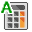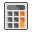RSWebinar: Designing Cost-Effective Ground Improvement Solutions with Settle3 | June 7th, 2023 Register Now

# Probabilistic Analysis Overview

Random Variables

In a Probabilistic Analysis, you can define statistical distributions for input parameters (e.g. pillar length, pillar width, pillar height), to account for uncertainty in the values of input parameters. When the analysis is computed, this results in a Factor of Safety distribution from which a Probability of Failure (PF) is calculated.

The general procedure for carrying out a Probabilistic Analysis with CPillar is as follows.

## 1. Select Analysis Type, Pillar Shape, and Analysis Method

In the Project Settings dialog:

• Set the Analysis Type to Probabilistic.
• Set the Pillar Shape to either Rectangular Pillar or Polygonal Pillar.
• Set the Analysis Method to one of the following:
• Rigid (Polygonal Pillar or Rectangular Pillar)
• Elastic (Rectangular Pillar)
• Voussoir (Rectangular Pillar)

## 2. Select Sampling Options

In the Project Settings dialog:

• Set the Sampling Method to one of the following:
• Monte Carlo
• Latin Hypercube
• Set the Number of Samples.
• Set the Design Factors of Safety (Shear, Elastic Buckling, Compression) and Buckling Parameter.

## 3. Select Random Numbers Options

In the Project Settings dialog:

• Set the Random Number Generation method to one of the following:
• Pseudo-random (and optionally Specify Seed)
• Random
• Set the Number Generator.

## 4. Defining Random Variables

In order to carry out a Probabilistic Analysis, you must define one or more input parameters (e.g. pillar length, pillar width, etc.) as random variables, by assigning statistical distributions to the desired variables and entering the required statistical parameters (e.g. mean, standard deviation). See the Random Variables topic for a summary of the random variables which can be defined in CPillar.

NOTE: Any input parameter which is NOT assigned a Statistical Distribution, is assumed to be exactly known (by the Mean value), and will not be involved in the statistical sampling process.

## 5. Compute

• If the Autocomputeoption is turned ON, then the Probabilistic Analysis is computed when you change the input data.
• If the Autocomputeoption is turned OFF, then the Probabilistic Analysis will need to be manually computed by selecting Computeafter you change the input data.
By default, Autocompute is turned ON.

## 6. Analysis Results

After the analysis is computed, the Probability of Failure will be displayed in the Pillar Information panel of the Sidebar. Detailed results are reported in the Info Viewer. Probabilistic input and output data can be plotted on a Histogram Plot, Scatter Plot, or Cumulative Plot. Data can also be exported for further analysis and processing.